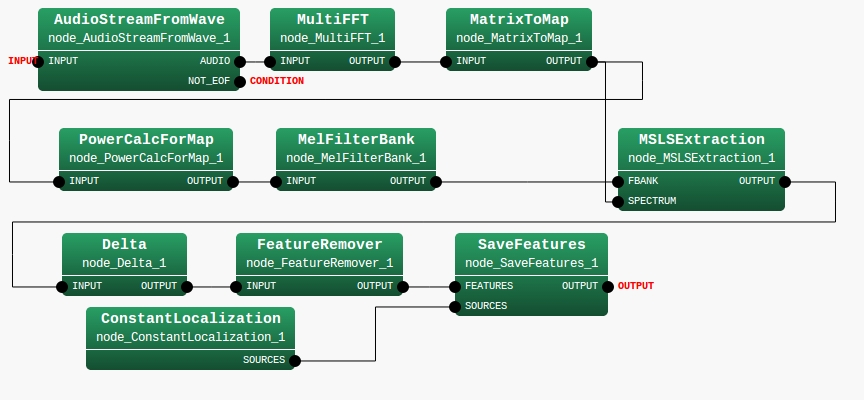## 14.5.6 MSLS+$\Delta$MSLS+$\Delta$Power

An execution example is shown in Figure 14.26. After the execution, a file named MFBANK27_0.spec is generated. This file stores little endian 27 dimensional vector sequence expressed in the 32 bit floating-point number format. When feature extraction cannot be performed well, check if the f101b001.wav files are in the data directory.

> ./demo.sh 5
UINodeRepository::Scan()
Scanning def /usr/lib/flowdesigner/toolbox
done!
Building network :MAIN

Figure 14.26: Execution example

Thirteen modules are included in this sample. There are three modules in MAIN_LOOP (iterator) and ten modules in MAIN (subnet). MAIN (subnet) and MAIN_LOOP (iterator) are shown in Figures 14.27 and 14.28 As an outline of the processing, it is simple network configuration in which acoustic features are calculated in MSLSExtraction with the audio waveforms collected in the AudioStreamFromWave module and are written in SaveFeatures . Since MSLSExtraction requires the outputs of the mel-scale filter bank and power spectra for calculation of MSLS, the collected audio waveforms are analyzed by MultiFFT and their data type are converted by MatrixToMap and PowerCalcForMap , and then processing to obtain outputs of the mel-scale filter bank is performed by MelFilterBank . MSLSExtraction reserves a storing region for the $\delta$ MSLS coefficient other than the MSLS coefficient and outputs vectors as a feature (zero is in the storing region for the$\delta$ MSLS coefficient). Since the USE_POWER property is set to true, a storing region of $\delta$ MSLS and the delta power term is secured for the $\delta$ coefficient.

Therefore, vectors that are double of the values specified in the FBANK_COUNT property of MSLSExtraction +1 are output as a feature. The $\delta$ MSLS coefficient and delta power term are calculated and stored with Delta . Since necessary coefficients are the MSLS coefficient and $\delta$ MSLS coefficient and delta power term, it is necessary to delete unnecessary power terms. Use FeatureRemover to delete them. SaveFeatures saves the input FEATURE. The localization result from the front generated by ConstantLocalization is gave to SOURCES.Figure 14.27: MAIN (subnet)Figure 14.28: MAIN_LOOP (iterator)

Table 14.17 summarizes the main parameters.

Table 14.17: Parameter list
 Node name Parameter name Type Value MAIN_LOOP LENGTH int :ARG2 ADVANCE int :ARG3 SAMPLING_RATE int :ARG4 FBANK_COUNT int :ARG5 FBANK_COUNT1 int :ARG6 DOWHILE (empty) FBANK_COUNT FBANK_COUNT NORMALIZE_MODE Cepstral USE_POWER true FBANK_COUNT1 FBANK_COUNT1 SELECTOR